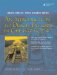# Streams

Streams are objects used for reading and writing. The Standard Library defines , while Qt defines <QTextStream> for the equivalent functionality.

iostream defines the three global streams:

• cin standard input (keyboard)
• cout standard output (console screen)
• cerr standard error (console screen)

Also defined (in both and ) are manipulators, such as flush and endl. A manipulator can be added to

• An output stream to change the way the output data is formatted
• An input stream to change the way that the input data is interpreted

The code in Example 1.15 demonstrates the use of several manipulators applied to the standard output stream.

Example 1.15. src/stdstreams/streamdemo.cpp

 ```#include int main() { using namespace std; int num1(1234), num2(2345) ; cout << oct << num2 << ' ' << hex << num2 << ' ' << dec << num2 << endl; cout << (num1 < num2) << endl; cout << boolalpha << (num1 < num2) << endl; double dub(1357); cout << dub << ' ' << showpos << dub << ' ' << showpoint << dub << endl; dub = 1234.5678; cout << dub << ' ' << fixed << dub << ' ' << scientific << dub << ' ' << noshowpos << dub << endl; } Output: 4451 929 2345 1 true 1357 +1357 +1357.00 +1234.57 +1234.567800 +1.234568e+03 1.234568e+03 ```

Streams are used for reading from or writing to files, network connections, and also strings. One useful feature of streams is that they make it easy to produce strings from mixed types of data. In Example 1.16, we will create some strings from numerics and write them to a file.

Example 1.16. src/stl/streams/streams.cpp

 ```[ . . . . ] int main() { using namespace std; ostringstream strbuf; int lucky = 7; float pi=3.14; double e=2.71; /* An in-memory stream */ strbuf << "luckynumber " << lucky << endl << "pi " << pi << endl << "e " << e << endl; string strval = strbuf.str(); <-- 1 cout << strval; /* An output file stream. */ ofstream outf; outf.open("mydata"); <-- 2 outf << strval ; outf.close(); ```   (1)convert the stringstream to a string (2)creates (or overwrites) a disk file for output

After the strings have been written, we have a couple of choices for how to read them. We can use the analogous input operators that we wrote to output, and because there is whitespace between each record, the insertion operator will work as shown in Example 1.17.

Example 1.17. src/stl/streams/streams.cpp

 ```[ . . . . ] /* An input file stream */ ifstream inf; inf.open("mydata"); string newstr; int lucky2; inf >> newstr >> lucky2; if (lucky != lucky2) cerr << "ERROR! wrong lucky number" << endl; float pi2; inf >> newstr >> pi2; if (pi2 != pi) cerr << "ERROR! Wrong pi." << endl; double e2; inf >> newstr >> e2; if (e2 != e) cerr << "e2: " << e2 << " e: " << e << endl; inf.close(); ```

In addition, we can read files line-by-line and deal with each line as a string, as shown in Example 1.18.

Example 1.18. src/stl/streams/streams.cpp

 ```[ . . . . ] /* Read line-by-line */ inf.open("mydata"); while(not inf.eof()) { getline(inf, newstr); cout << newstr << endl; } inf.close(); return 0; } ```

Exercise: Streams

Modify the program in Example 1.16 so that it does the following:

• It gets the file name from the user as an STL string fileName. You will need to use the function fileName.c_str() to convert the string to a form that is acceptable to the open( ) function.
• It makes sure that the file specified by the user does not already exist (or that it is all right to overwrite it if it does exist) before opening it for output.
• It makes sure that the file exists before attempting to read from it. (Hint: After the call to open, you can test the ifstream variable as if it were a bool. false means that the file does not exist.)An Introduction to Design Patterns in C++ with Qt 4
ISBN: 0131879057
EAN: 2147483647
Year: 2004
Pages: 268